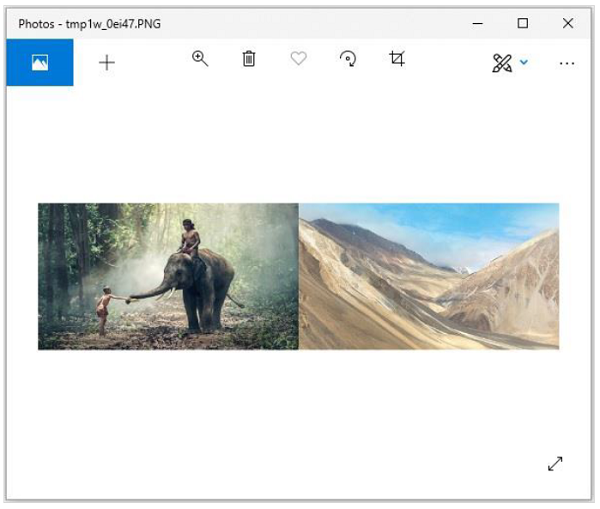# Python Pillow - Merging Images

Pillow package allows you to paste an image onto another one. The merge() function accepts a mode and a tuple of images as parameters, and combines them into a single image.

### Syntax

```Image.merge(mode, bands)
```

Where,

• mode − The mode to use for the output image.

• bands − A sequence containing one single-band image for each band in the output image. All bands must have the same size.

• Return value − An Image objects.

Using the merge() function, you can merge the RGB bands of an image as −

```from PIL import Image
image = Image.open("beach1.jpg")
r, g, b = image.split()
image.show()
image = Image.merge("RGB", (b, g, r))
image.show()
```

On executing the above piece of code, you can see the original image and the image with merge the RGB bands as shown below −

Input image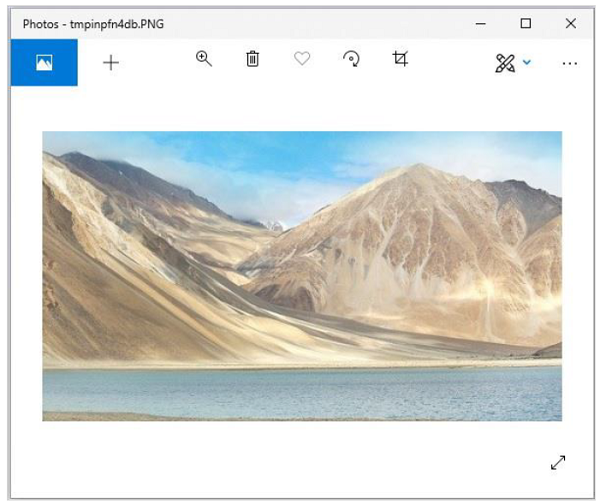Output image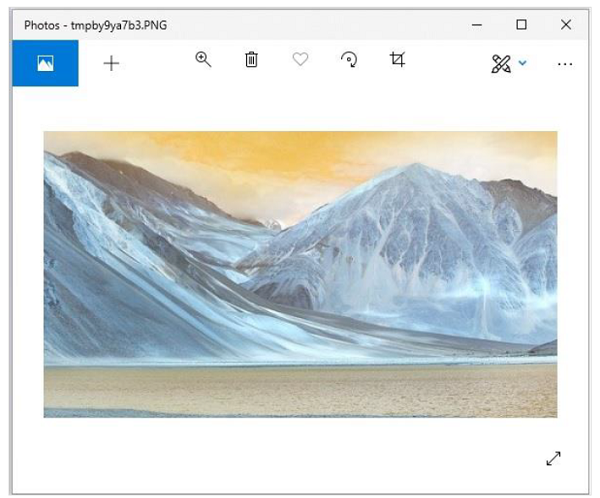### Merging two images

In the same way, to merge two different images, you need to −

• Create image object for the required images using the open() function.

• While merging two images, you need to make sure that both images are of same size. Therefore, get each sizes of both images and if required, resize them accordingly.

• Create an empty image using the Image.new() function.

• Paste the images using the paste() function.

• Save and display the resultant image using the save() and show() functions.

### Example

Following example demonstrates the merging of two images using python pillow −

```from PIL import Image
image1 = Image.open('images/elephant.jpg')
image1.show()
image2.show()
#resize, first image
image1 = image1.resize((426, 240))
image1_size = image1.size
image2_size = image2.size
new_image = Image.new('RGB',(2*image1_size, image1_size), (250,250,250))
new_image.paste(image1,(0,0))
new_image.paste(image2,(image1_size,0))
new_image.save("images/merged_image.jpg","JPEG")
new_image.show()
```

### Output

If you save the above program as Example.py and execute, it displays the two input images and the merged image using standard PNG display utility, as follows −

Input image1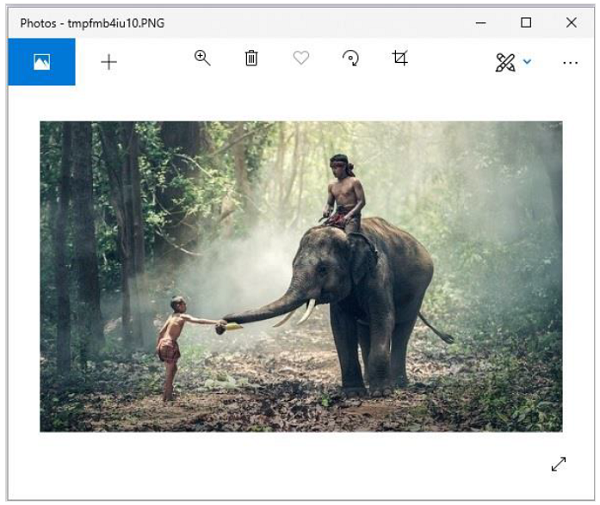Input image2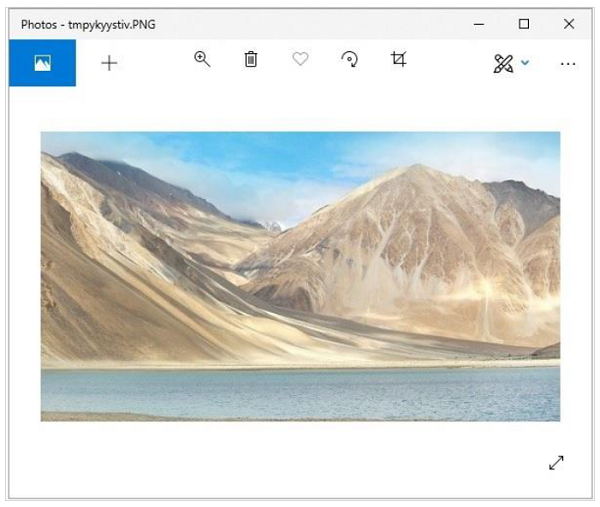Merged image